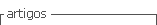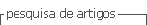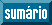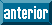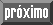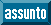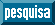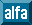## Artigo

•Citado por SciELO
•Acessos

•Citado por Google
•Similares em SciELO
•Similares em Google

## versão impressa ISSN 0120-419X

### Integración - UIS vol.35 no.2 Bucaramanga jul./dez. 2017

#### http://dx.doi.org/10.18273/revint.v35n2-2017005

Artículos originales

Approximation properties on Herz spaces

Propiedades de aproximación en espacios de Herz

Jhean E. Pérez-López1

1Universidad Industrial de Santander, Escuela de Matemáticas, Bucaramanga, Colombia

Abstract

In this paper we consider the Herz spaces Kα p,q , which are a natural generalization of the Lebesgue spaces Lp . We prove some approximation properties such as density of the space C c (R n ), continuity of the translation, continuity of the mollification, global behavior of the convolution with smooth functions, among others.

MSC2010: 26B05, 26B35, 26B99.

Key words: Herz spaces; Mollifiers; Convolution; Functional spaces

Resumen

En este artículo consideramos los espacios de Herz Kα p,q , los cuales son una generalización natural de los espacios de Lebesgue Lp . Demostramos algunas propiedades de aproximación tal es como densidad del espacio C c (R n ), continuidad de la traslación, continuidad de la molificación, comportamiento global de la convolución con funciones suaves, entre otras.

Palabras-clave: Espacios de Herz; Molificadores; Convolución; Espacios funcionales

1. Introduction

The Herz space Kα p,q was introduced by Herz  as a suitable environment for the image of the Fourier transform acting on a class of Lipschitz spaces, in order to obtain a Bernstein type theorem. A characterization of the Kα p,q -norm in terms of Lp -norms over annuli was given in  (see Definition 2.1). Recently, some versions of classical spaces based on Herz spaces Kα p,q , such as Hardy-Herz, Sobolev-Herz and Triebel-Lizorkin-Herz spaces, have presented an increasing interest in the literature of function spaces and turned out to be a useful tool in harmonic analysis (see ,,, and references therein). We also refer the reader to  for results of well-posedness of the Navier-Stokes equations in weak-Herz spaces, and to  for results of well-posedness of the Euler equations on Besov-Herz spaces.

In this paper we consider the Herz spaces Kαp,q, which are a natural generalization of the Lebesgue spaces Lp . We prove some approximation properties such as density of the spaces C c (R n ), continuity of the translation, continuity of the mollification, global behavior of the convolution with smooth functions, among others. This results generalize the corresponding ones in Lp . They seem necessary for the study of the transport equations as done in  in the frame work of the Lp spaces.

This paper is organized as follows. In Section 2 we recall the definition of the Herz spaces Kα p,q and some basic properties. Section 3 is devoted to the new results about approximation and global behavior of functions in Herz spaces and his convolution with smooth functions.

2. Herz spaces

This section is devoted to recall the definition of Herz spaces and establish some of their properties, which will be useful in the remainder of the paper.

For k integer and k ≥ −1, let Ak be defined as

where B(x 0,R) = {x ∈ R n ; |xx 0| < R}. Then we have the disjoint decomposition R n = ∪ k≥−1 Ak .

Definition 2.1. Let 1 ≤ p,q ≤ ∞ and α ∈ R. The Herz space Kα p,q = Kα p,q (R n ) is defined by

where

In the case p = 1, we consider Kα p,q as a space of signed measures, with ║fL¹(Ak) denoting the total variation of f on Ak . The pair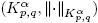is a Banach space for α ∈ R, 1 ≤ p < ∞ and 1 ≤ q ≤ ∞ (see e.g. ). Also, note that K 0 p,1Lp = K 0 p,p K 0 p,∞. So, all the results presented here are a generalization of the corresponding results in Lp . There is a version of Hölder’s inequality in Herz spaces (see ). In fact, let 1 ≤ p, p 1, p 2, q, q 1, q 2 ≤ ∞ and α, α 1, α 2 ∈ R be such that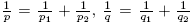, and α = α 1 + α 2. Then,

In particular,

The following result provides a control in Kα p,q -spaces for the action of a volume preserving diffeomorphism. Recall that a diffeomorphism X is volume preserving if for all measurable set Ω, we have μ(Ω) = μ(X(Ω)), where μ represent the Lebesgue measure.

Lemma 2.2 (). Let 1 ≤ p, q ≤ ∞ and α ≥ 0. Let X: R n → R n be a volume-preserving diffeomorphism such that, for some fixed ω > 0,

where |·| stands for the Euclidean norm in R n. Then, there exists C > 0 such that

for all fKα p,q .

The next lemma is key in order to prove the new results in Section 3. It provides an estimate for convolution operators in Herz spaces depending on certain weighted norms of the kernel θ.

Lemma 2.3 (). (Convolution). Let α ∈ R and 1 ≤ p, q ≤ ∞. Let θL 1 be such that Mθ < ∞ for some β > 0, where

Then, there is a constant C > 0 (independent of θ) such that

To finish this section we present a first result of density in Herz spaces.

Lemma 2.4 (). Let 1 ≤ p,q ≤ ∞ and α ∈ R . Then, Schwartz space S is continuously included in Kα p,q. Moreover, the inclusion is dense provided that 1 ≤ p,q < ∞.

3. New results

Now we present some new results about approximation of functions in Herz spaces and global behavior of their convolution with smooth functions. This results can be useful in order to analyze the well-posedness of some PDEs in the framework of Herz spaces.

In what follows, let ρS such that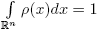. For > 0 we define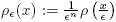, additionally, for a mensurable function f ∈ Rn → R we also define f∊ (x) = (ρ∊f) (x).

We first show that not only S but also C c (R n ) are dense in Herz spaces.

Theorem 3.1. Let 1 ≤ p,q < ∞ and α ∈ R. Then, the space C c (R n ) is dense in Kα p,q .

Proof. From Lemma 2.4 it follows that S is dense in Kα p,q . Let fKα p,q, ∊ > 0 and φS such that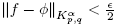. Now, let N ∈ N such that

and let θC c (R n ) be such that 0 ≤ θ ≤ 1, θ = 1 in B (0, 2 N ) and θ = 0 in [B (0, 2 N )] c . Defining ψ = θφ, we have that ψC c (R n ), ψ = φ in B (0, 2 N ) and θ = 0 in [B (0, 2 N )] c . Moreover, it follows that

Thus,

Since > 0 is arbitrary, we conclude the proof. ☑

Now we prove the continuity of the translation mapping in Herz spaces.

Theorem 3.2. Let 1 ≤ p,q < ∞ and α ∈ R. Then, τzff in Kα p,q as z → 0 for all f ∈ Kα p,q. Here τzf denotes the mapping such that τzf(x) = f(xz).

Proof. Note that, for |z| < 1, we have that the volume preserving diffeomorphism Xz (x) = xz trivially verifies ║Xz Id║∞ ≤ 1. Now, let f ∈ Kα p,q , ∞ > 0 and g ∈ C c (R n ) such that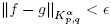. Using Lemma 2.2, it follows that

Now we prove that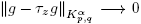when z → 0. In fact, if |z| < 1, then suppg and suppτzg are contained in a common compact set K. Choosing M ∈ N such that KB (0, 2 M ), and using that τzgg in Lp (R n ) , we have that

Thus, taking lim sup as z → 0 in (9) and using (10) we obtain

since > 0 is arbitrary, we obtain the result. ☑

Lemma 3.3. Let 1 ≤ p,q < ∞, α ∈ R, fKα p,q and ρ ∈ S. Then,

where 0 < C(, ρ) ≤ C˜ (ρ) if 0 < < 1.

Proof. Using Lemma 2.3 we have that

where

Moreover, if 0 < < 1 is easy to see that Mρ∊ Mρ . ☑

Now we show the continuity of the mollification of a function in Herz spaces when the mollifier is in C c (R n ).

Theorem 3.4. Let fKα p,q with 1 ≤ p,q < ∞, α ∈ R and ρC c (R n ). Then, f f in Kα p,q as → 0.

Proof. By definition we have that

where we use the change y = ∊z. Now, using Minkowski’s inequality we get

Thus, using again Minkowski’s inequality (for sequences) we obtain

where suppρ = K. Since K is compact, we have that |z| < C 2 for all zK; moreover, for 0 < ≪ 1 we also have that |∊z| < 1 for all zK. Thus, using Lemma 2.2 we have that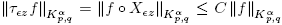and it follows that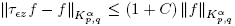. Then, using (11), Proposition 3.2 and the Dominate Convergence Theorem we obtain the result. ☑

The following is an auxiliary lemma.

Proposition 3.5. Let φC c (R n ), 1 ≤ p,q ≤ ∞, α ∈ R and ρS. Then, φ φ in Kα p,q as ∊ → 0.

Proof. Let N ∈ N such that suppφB(0, 2 N ), and let M > N + 2. For kM and xAk we have that

Thus, for any s > 0 we get

On the other hand,

Is clear that S 1 → 0 as → 0, because ρ φφ in Lp (Ak ). For S 2, using the Hölder inequality, (12) and taking s > 0 large enough, we have

Then, S 2 → 0 as → 0. The estimates for S 1 and S 2 give the result. ☑

Now we prove the continuity of the mollification of a functions in Herz spaces when the mollifier is in S.

Theorem 3.6. Let fKα p,q with 1 ≤ p,q < ∞, α ∈ R and ρS. Then, f f in Kα p,q as → 0.

Proof. Let δ > 0 and φC c (R n ) such that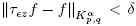. For 0 < ≪ 1, we have

Taking → 0 and using Lemma 3.5, we obtain

Since δ > 0 was arbitrary, we prove the proposition. ☑

The following two results are related to the global behavior of convolutions of functions in Herz spaces with smooth functions.

Theorem 3.7. Let 1 ≤ p, q ≤ ∞, α ≥ 0, fKα p,q and ψC c . Then fψC (R n ) and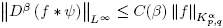for all β multi-index.

Proof. Note that fψC (R n ) follows from the fact that fL 1 loc and the classic theory of convolution. On the other hand, by definition we have

Let N ∈ N such that suppψB(0, 2 N ), and note that

Thus, if |x| ≤ 2 N+1, then (Ak−{x})∩suppψ = ∅ for kN +3; therefore, for |x| ≤ 2 N+1 we have

On the other hand, if |x| > 2 N+1, it follows that,

and

Then,

Thus, for any x ∈ R n we obtain

Similarly, for any x ∈ R n we have

Theorem 3.8. Let 1 ≤ p,q < ∞, α ≥ 0, fKα p,q and ψC c (R n ). Then fψC 0 (R n ) .

Proof. Let ∊ > 0 and φC c (R n ) such that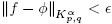. Then,

Since φ, ψC c (R n ), we have that φψC c (R n ); thus, |x| > C = C(), and we have that (φψ) (x) = 0. Therefore, for x large enough, it follows that

Now, proceeding as in the proof of the Theorem 3.7, we get

Since > 0 is arbitrary, we get the result. ☑

References

 Chen Y.Z. and Lau K.S., “On some new classes of Hardy spaces”, J. Funct. Anal. 84 (1989), 255–278. [ Links ]

 DiPerna R.J. and Lions P.L., “Ordinary differential equations, transport theory and Sobolev spaces”, Invent. Math. 98 (1989), No. 3, 511–547. [ Links ]

 Ferreira L.C.F. and Pérez-López J.E., “On the theory of Besov–Herz spaces and Euler equations”, Israel J. Math. 220 (2017), No. 1, 283–332. [ Links ]

 García-Cuerva J. and Herrero M.-J.L., “A theory of Hardy spaces associated to the Herz spaces”, Proc. Lond. Math. Soc. (3) 69 (1994), No. 3, 605–628. [ Links ]

 Grafakos L., Li X. and Yang D., “Bilinear Operators on Herz-type Hardy spaces”, Trans. Amer. Math. Soc. 350 (1998), No. 3, 1249–1275. [ Links ]

 Hernandez E. and Yang D., “Interpolation of Herz spaces and applications”, Math. Nachr. 205 (1999), No.1, 69–87. [ Links ]

 Herz C.S., “Lipschitz spaces and Bernstein’s theorem on absolutely convergent Fourier transforms”, J. Math. Mech. 18 (1968/69), 283–323. [ Links ]

 Johnson R., “Lipschitz spaces, Littlewood-Paley spaces, and convoluteurs”, Proc. Lond. Math. Soc. (3) 29 (1974), No. 1, 127–141. [ Links ]

 Tsutsui Y., “The Navier-Stokes equations and weak Herz spaces”, Adv. Differential Equations 16 (2011), No. 11-12, 1049–1085. [ Links ]

 Xu J., “Equivalent norms of Herz-type Besov and Triebel-Lizorkin spaces”, J. Funct. Spaces Appl. 3 (2005), No. 1, 17–31. [ Links ]

Received: August 11, 2017; Accepted: December 14, 2017This work is under License Creative Commons Attribution 4.0 International.